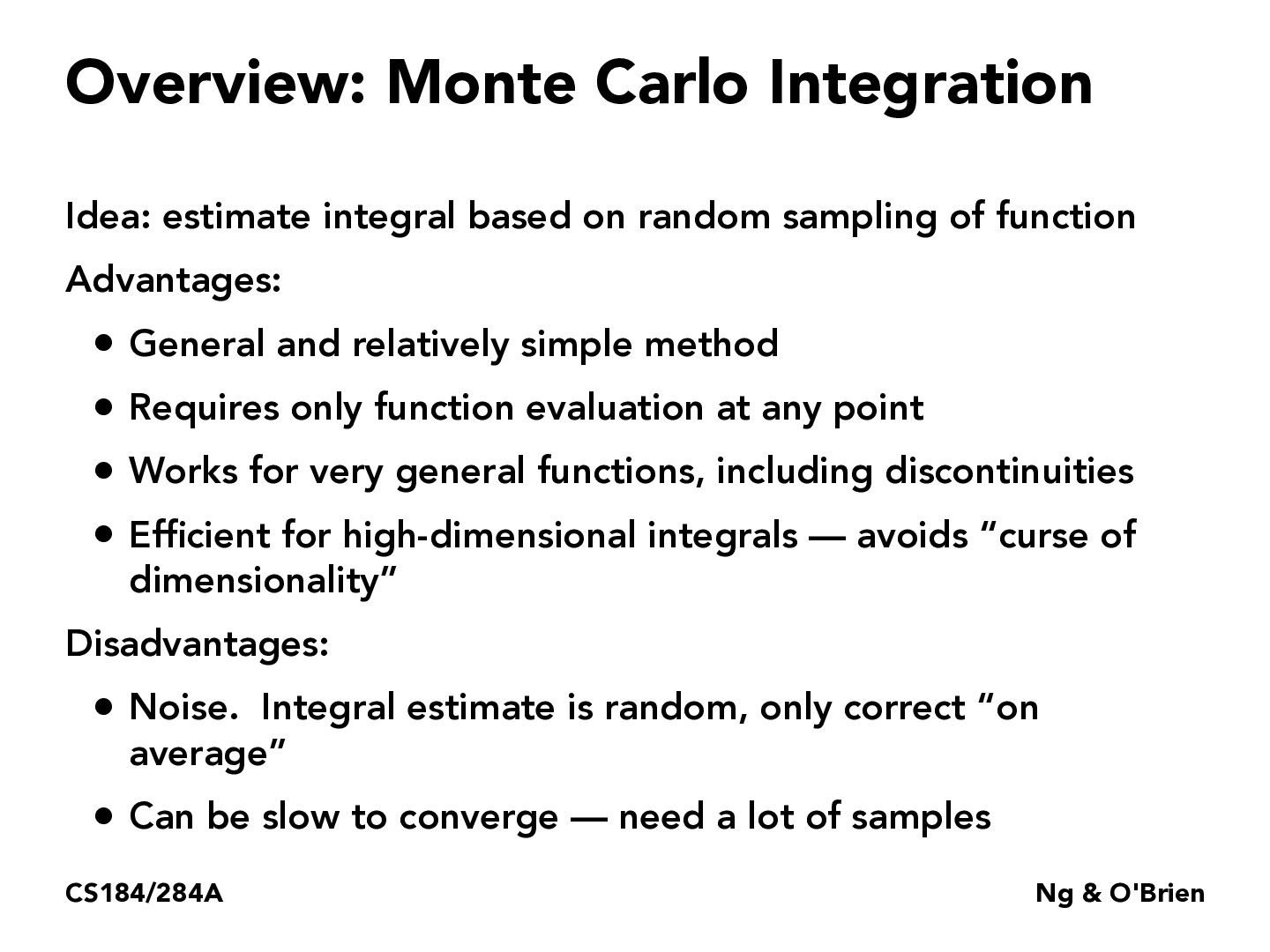Lecture 12: Monte Carlo Integration (12)countermoe

I know that denoising is an important process in speeding up some of the computation that comes with global illumination. At what point is it most effective to switch to an algorithm that "fills in the gaps" from the noise? What are the trade-offs that come from something like this?ld184

how is convergence defined here? when would we consider a result "converged"?Spectato54

In what sense is Monte Carlo is being applied in graphics? Is it for modeling textures such as fluid dynamics or more molecular modeling such as the interactions of a light particle? Maybe both?Monte Carlo Integration is a technique used to address the "curse of dimensionality" which affects numerical integration. slide 9 suggests Monte Carlo Integration may be useful for global illumination. Slide 31 shows an example of using the technique to calculate irradiance.Unicorn53547

Monte Carlo is widely used in rendering a 3D scene by randomly tracing samples of possible light paths. Repeated sampling of any given pixel will eventually cause the average of the samples to converge on the correct solution of the rendering equation, making it one of the most physically accurate 3D graphics rendering methods in existence. A quick intro and some examples can be seen here